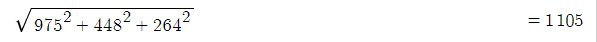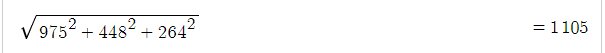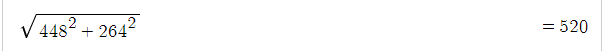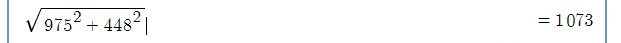# Could this be a Perfect Euler Brick?

alpha_michi
so here is the Math:

for a²+b²:for b²+c²:
https://www.physicsforums.com/attachments/284600
for a²+b²+c²:alpha_michi
SORRY here:Staff Emeritus
Gold Member
You almost had me. I didn't know what this was, but after seeing it on Wikipedia thought there was no way someone didn't try all the integers smaller than a thousand already.

Anyway, you forgot one of the face diagonals. ##\sqrt{975^2+264^2}## is not an integer.

alpha_michi
You almost had me. I didn't know what this was, but after seeing it on Wikipedia thought there was no way someone didn't try all the integers smaller than a thousand already.

Anyway, you forgot one of the face diagonals. ##\sqrt{975^2+264^2}## is not an integer.
Ok, I will try that one too.

Mentor
Yes, there has been an extensive search with quite a large range of numbers and sadly no discovery yet nor even an impossibility proof.

•Delta2
aheight
Regarding the Wikipedia reference: Results of computer search for a perfect cuboid:

A suite of optimized computer programs was designed to systematically search for a perfect cuboid, keep track of close misses, and investigate the statistical trend of these near matches with increasing Euler brick size. While no perfect cuboid was found, the minimum length of the odd side has been substantially extended from the prior published limit of 3 trillion1 (3 x 10^12) to 25 trillion (2.5 x 10^13), and the minimum side has increased from the prior published limit of 10 billion2 (10^10) to 500 billion (5 x 10^11 ).

The author concludes:

While some interesting near-misses have been identified, the overall trend with increasing minimum side does not favor the existence of a perfect cuboid.
This seems naive to suggest after only checking a basically insignificant number of cases, i.e., there may be one that has extremely large faces. Why would he make what I consider to be a foolish claim?

Mentor
More often than not, researchers do not make claims they can’t support. The search for a perfect Euler brick has been done to a great degree.

While it’s truly a small percentage of an infinitude of possible numbers, I suspect a sequence of solutions indicates that the near misses are happening less often and perhaps with greater differences in the near miss category that indicates we may never find a solution.

Also I’m sure they’ve published a paper somewhere that describes the background of their conclusion and it would be worthwhile to investigate further.

•pbuk
Homework Helper
Gold Member
Also I’m sure they’ve published a paper somewhere that describes the background of their conclusion and it would be worthwhile to investigate further.
Yes, this is the paper linked and quoted from in #6 and this does indeed describe two reasons for Matson's claim which @aheight seems to have overlooked:

A perhaps more telling trend is the plot of least-significant matching bit (where all higher bits match). As X increases, the point of Y2 mismatch (reading from most-significant to least-significant bit) is rising at nearly the same rate as the number of bits in X. This trend suggests that it is highly unlikely that there is a perfect cuboid.​

and

A plot of the results for the even side X cases up to 200 billion is shown below, followed by the corresponding plot of least-significant matching bit (where all higher bits match). As with the odd side plot, the point of Y2 mismatch is rising at about the same rate as the number of bits in X, again suggesting poor odds that there is a perfect cuboid.​

Mentor
As an aside, I played with this problem once on my iPad using Pythonista's python.

Basically, I generated a table of squares, to create a table of Pythagorean triples and then started a search for matching triples ie ones with common square components.

It was a fun project, but of course the iPad could never compete with a super computer in searching a vast range of possibilities.

aheight
Yes, this is the paper linked and quoted from in #6 and this does indeed describe two reasons for Matson's claim which @aheight seems to have overlooked:

A perhaps more telling trend is the plot of least-significant matching bit (where all higher bits match). As X increases, the point of Y2 mismatch (reading from most-significant to least-significant bit) is rising at nearly the same rate as the number of bits in X. This trend suggests that it is highly unlikely that there is a perfect cuboid.​

and

A plot of the results for the even side X cases up to 200 billion is shown below, followed by the corresponding plot of least-significant matching bit (where all higher bits match). As with the odd side plot, the point of Y2 mismatch is rising at about the same rate as the number of bits in X, again suggesting poor odds that there is a perfect cuboid.​
Ok, thanks for that. Sorry I missed it. I'm not a Number theorist but am personally skeptical we can predict the future behavior of diophantine expressions based on the previous results of those expressions. My understanding is that integer arithmetic does not work this way. Rather it is discontinuous and often involves abrupt changes. For example a number N can have a hundred factors but N+1 can be prime. Is this a flawed understanding?

••